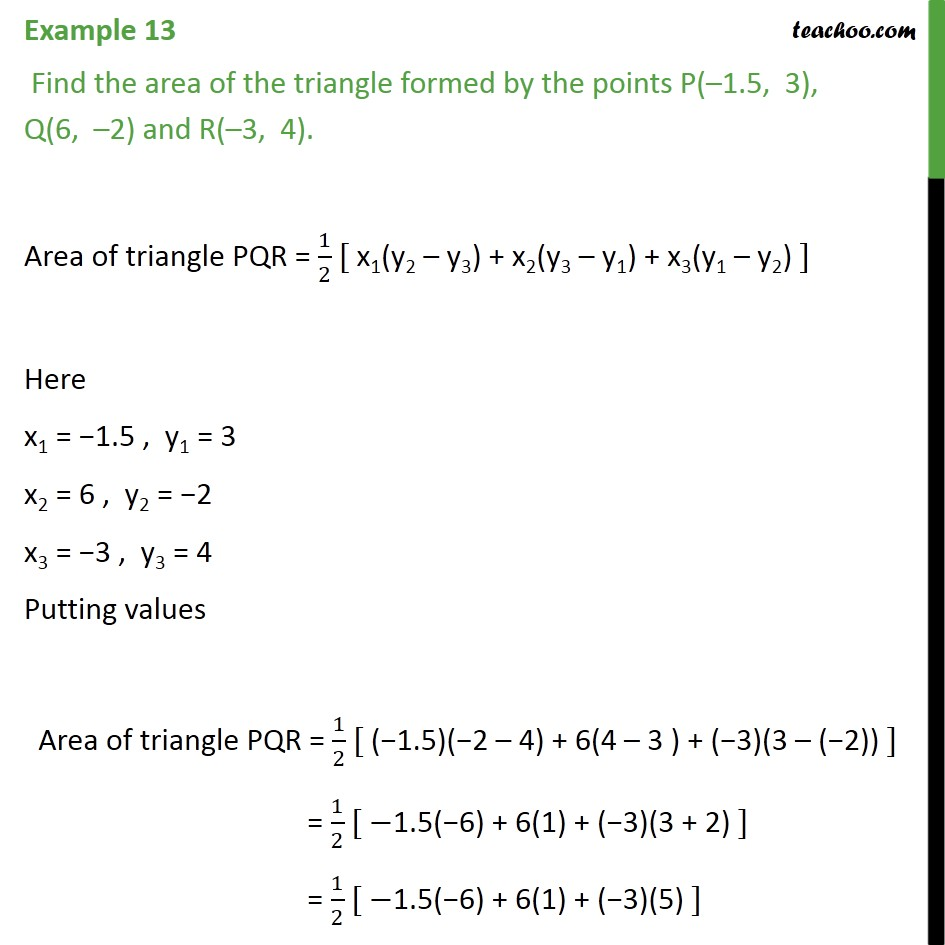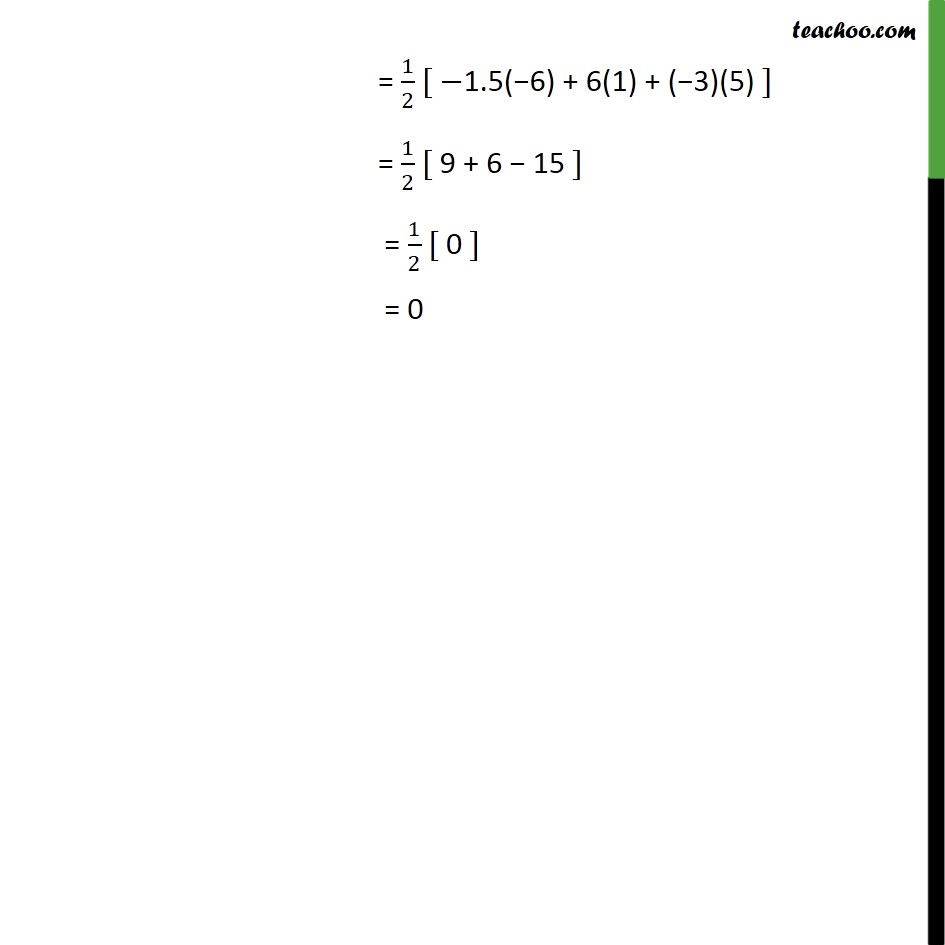1. Chapter 7 Class 10 Coordinate Geometry
2. Serial order wise
3. Examples

Transcript

Example 13 Find the area of the triangle formed by the points P( 1.5, 3), Q(6, 2) and R( 3, 4). Area of triangle PQR = 1/2 [ x1(y2 y3) + x2(y3 y1) + x3(y1 y2) ] Here x1 = 1.5 , y1 = 3 x2 = 6 , y2 = 2 x3 = 3 , y3 = 4 Putting values Area of triangle PQR = 1/2 [ ( 1.5)( 2 4) + 6(4 3 ) + ( 3)(3 ( 2)) ] = 1/2 [ 1.5( 6) + 6(1) + ( 3)(3 + 2) ] = 1/2 [ 1.5( 6) + 6(1) + ( 3)(5) ] = 1/2 [ 1.5( 6) + 6(1) + ( 3)(5) ] = 1/2 [ 9 + 6 15 ] = 1/2 [ 0 ] = 0

Examples